# A. Xu Xiake in Henan Province

## Description:

Shaolin Monastery, also known as the Shaolin Temple, is a Chan ("Zen") Buddhist temple in Dengfeng County, Henan Province. Believed to have been founded in the $5$-th century CE, the Shaolin Temple is the main temple of the Shaolin school of Buddhism to this day.
Longmen Grottoes, are some of the finest examples of Chinese Buddhist art. Housing tens of thousands of statues of Buddha and his disciples, they are located $12$ kilometres ($7.5$ mi) south of present-day Luoyang in Henan province.
White Horse Temple is, according to tradition, the first Buddhist temple in China, established in $68$ AD under the patronage of Emperor Ming in the Eastern Han dynasty capital Luoyang.
The Yuntai Mountain is situated in Xiuwu County, Jiaozuo, Henan Province. The Yuntai Geo Park scenic area is classified as an AAAAA scenic area by the China National Tourism Administration. Situated within Yuntai Geo Park, with a fall of 314 metres, Yuntai Waterfall is claimed as the tallest waterfall in China.
They are the most famous local attractions in Henan Province.Now it's time to estimate the level of some travellers. All travellers can be classified based on the number of scenic spots that have been visited by each of them.
A traveller that visited exactly $0$ above-mentioned spot is a "Typically Otaku". A traveller that visited exactly $1$ above-mentioned spot is a "Eye-opener". A traveller that visited exactly $2$ above-mentioned spots is a "Young Traveller". A traveller that visited exactly $3$ above-mentioned spots is a "Excellent Traveller". A traveller that visited all $4$ above-mentioned spots is a "Contemporary Xu Xiake". Please identify the level of a traveller.

## Input:

The input contains several test cases, and the first line contains a positive integer $T$ indicating the number of test cases which is up to $10^4$.
For each test case, the only one line contains four integers $A_1$, $A_2$, $A_3$ and $A_4$, where $A_i$ is the number of times that the traveller has visited the $i$-th scenic spot, and $0 \leq A_1, A_2, A_3, A_4 \leq 100$. If $A_i$ is zero, it means that the traveller has never been visiting the $i$-th spot.

## Output:

For each test case, output a line containing one string denoting the classification of the traveller which should be one of the strings "Typically Otaku", "Eye-opener", "Young Traveller", "Excellent Traveller" and "Contemporary Xu Xiake" (without quotes).

5
0 0 0 0
0 0 0 1
1 1 0 0
2 1 1 0
1 2 3 4

## Sample Output:

Typically Otaku
Eye-opener
Young Traveller
Excellent Traveller
Contemporary Xu Xiake

## AC代码:

#include <bits/stdc++.h>
using namespace std;

const int maxn = 4;

int N;
int Cnt;
int A[maxn];

int main(int argc, char *argv[]) {
scanf("%d", &N);
for (int i = 0; i < N; ++i) {
Cnt = 0;
for (int j = 0; j < 4; ++j) {
scanf("%d", &A[j]);
if (A[j]) {
Cnt++;
}
}
if (!Cnt) {
printf("Typically Otaku\n");
}
else if (Cnt == 1) {
printf("Eye-opener\n");
}
else if (Cnt == 2) {
printf("Young Traveller\n");
}
else if (Cnt == 3) {
printf("Excellent Traveller\n");
}
else {
printf("Contemporary Xu Xiake\n");
}
}
return 0;
}

# D. Keiichi Tsuchiya the Drift King

## Description:

Drifting is a driving style in which the driver uses the throttle, brakes, clutch, gear shifting and steering input to keep the car in a state of oversteer while manoeuvring from turn to turn. As a sport, the drifting was first practised in Japan in the late 80s before gaining worldwide popularity in the decade that followed.
Keiichi Tsuchiya is a Japanese driver who is better known as the Drift King. He played an important role in popularizing the art of drifting. This pioneer inspired many successful drivers. He appeared in the movie The Fast and the Furious: Tokyo Drift and he is often employed on various movie sets as both driver and stunt coordinator. Keiichi Tsuchiya's talent in the drifting is especially true of his big stunt, the ultimate drifting.
Here is what he could do. The drift car he drives is shaped like a rectangular box of width $a$ inches and of length $b$ inches. He makes a right turn of a curve whose internal boundary is an arc with $d$ degrees in a circle with a radius of $r$ inches. As a super-skilled driver, he maintains his car to keep the contact and tangency at the internal boundary. That is, the right front corner of the car should always run along the internal boundary and the direction of the car body should always be tangential to the internal boundary.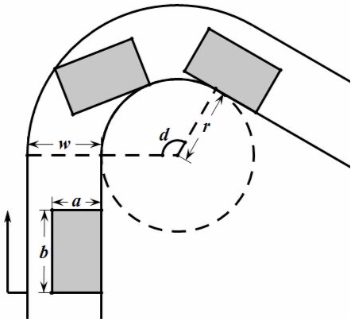We have measured that the straightaways before and after the curve are long enough, and the width of the lane is invariable. As what we meet in real life, if a lane has a fixed width, for each point of its one side, the distance to the nearest point of the other side is exactly its width. Now you are asked to calculate the minimal width $w$ of the lane so that the Drift King could drive throughout the curve without drifting out of the lane.

## Input:

The input contains several test cases, and the first line contains a positive integer $T$ indicating the number of test cases which is up to $10^4$.
For each test case, the only one line contains four integers $a$, $b$, $r$ and $d$, where $0 < a, b, r < 100$ and $0 < d < 180$.

## Output:

For each test case, output a line containing the minimal width (in inches) of the lane with an absolute or relative error of at most $10^{-6}$. Precisely speaking, assuming that your answer is $a$ and the jury's answer is $b$, your answer will be considered correct if $\frac{|a - b|}{\max\{1, |b|\}} \leq 10^{-6}$.

4
1 2 2 120
1 2 2 60
1 2 2 30
1 2 2 15

## Sample Output:

1.605551275464
1.605551275464
1.598076211353
1.415415569072

### 题目链接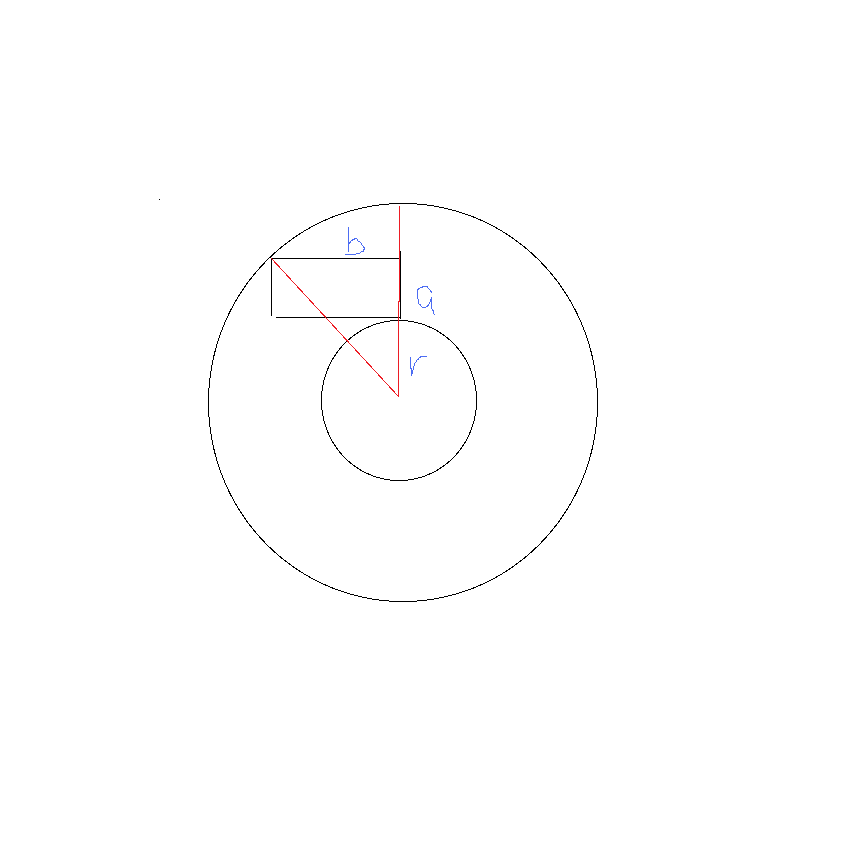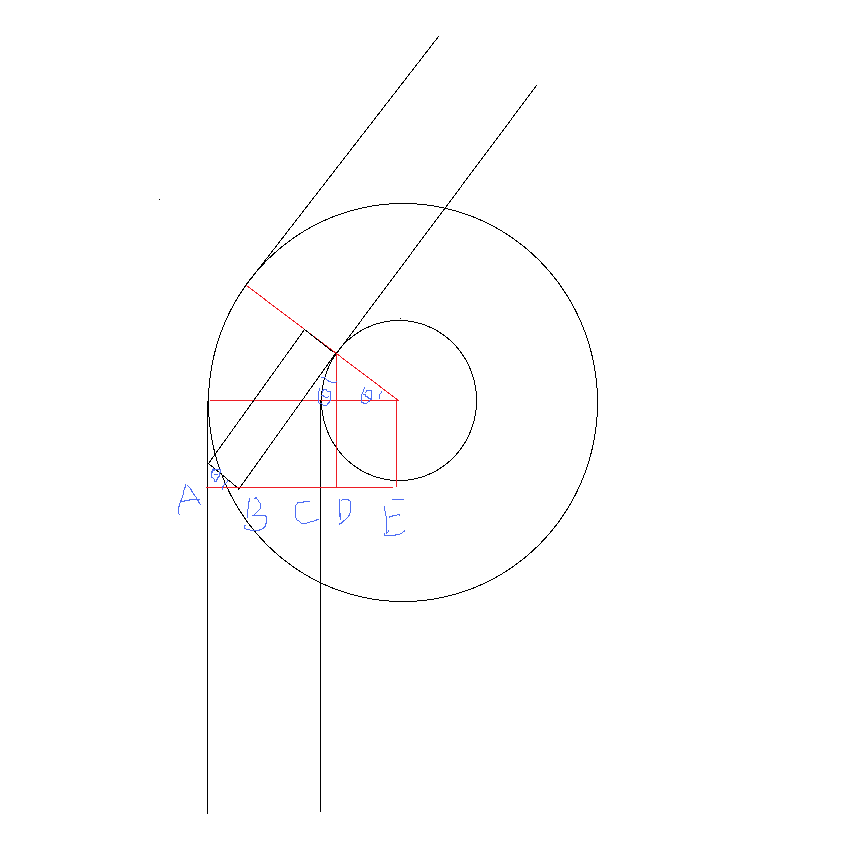## AC代码:

#include <bits/stdc++.h>
using namespace std;

const double pi = acos(-1.0);

int T;
double A, B, R, D;
double Tan;
double Sin, Cos;
double Ans;

int main(int argc, char *argv[]) {
scanf("%d", &T);
for (int Case = 1; Case <= T; ++Case) {
scanf("%lf%lf%lf%lf", &A, &B, &R, &D);
Tan = B / (A + R);
if (Tan < tan(D * pi / 180) || (Tan > 0 && tan(D * pi / 180) < 0)) {
Sin = B / sqrt(B * B + (A + R) * (A + R));
Cos = (A + R) / sqrt(B * B + (A + R) * (A + R));
}
else {
Sin = sin(D * pi / 180);
Cos = cos(D * pi / 180);
}
Ans = A * Cos + B * Sin + R * Cos - R;
printf("%.12lf\n", Ans);
}
return 0;
}

# E. Resistors in Parallel

## Description:

In this physics problem, what we are concerned about are only resistors. If you are poor at physics, do not worry, since solving this problem does not require you to have advanced abilities in physics.
Resistors are said to be connected together in parallel when both of their terminals are respectively connected to each terminal of the other resistors.We have the following parallel resistor equation for k resistors with resistances R1, R2, ..., Rk in parallel and their combined resistance R:

$$\displaystyle R = \frac{1}{\frac{1}{R_1} + \frac{1}{R_2} + \cdots + \frac{1}{R_k}}.$$

Now you have n resistors, the i-th of which has a resistance of ri ohms with the equation

$$\displaystyle r_i = \begin{cases} \infty &amp; \text{if i can be divided by d^2 for some integers d\ge 2,}\\ i &amp; \text{otherwise.} \end{cases}$$

You also have n selections, the i-th of which is a set of resistors Si such that

$$\displaystyle S_i = \{\text{the j-th resistor} \mid \text{j is a divisor of i}\}.$$

Please find a selection in which the resistors form a parallel resistor with the minimum resistance and output the reduced fraction of its resistance.

## Input:

The input contains several test cases, and the first line contains a positive integer T indicating the number of test cases which is up to 100.
For each test case, the only one line contains an integer n, where $1 ≤ n ≤ 10^{100}$.

## Output:

For each test case, output a line containing a reduced fraction of the form p/q indicating the minimum possible resistance, where p and q should be positive numbers that are coprime.

3
10
100
1000

1/2
5/12
35/96

## AC代码:

import math

maxn = 1005

def PrimeInit():
IsPrime = [True] * maxn
Prime = []
for i in range(2, maxn):
if IsPrime[i]:
Prime.append(i)
for j in range(i * i, maxn, i):
IsPrime[j] = False;
return Prime

def main():
Prime = PrimeInit()
T = int(input())
for Case in range(T):
N = int(input())
Book, Up, Down = 1, 1, 1
for i in Prime:
Book *= i
if Book > N:
break
Up *= i
Down *= i + 1
Gcd = math.gcd(Up, Down)
Up //= Gcd
Down //= Gcd
print(str(Up) + '/' + str(Down))

if __name__ == '__main__':
main()

# F. Honeycomb

## Description:

A honeycomb is a mass wax cells built by honey bees, which can be described as a regular tiling of the Euclidean plane, in which three hexagons meet at each internal vertex. The internal angle of a hexagon is $120$ degrees, so three hexagons at a point make a full $360$ degrees. The following figure shows a complete honeycomb with $3$ rows and $4$ columns.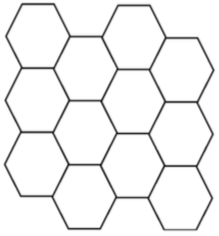Here we guarantee that the first cell in the second column always locates in the bottom right side of the first cell in the first column, as shown above. A general honeycomb may, on the basis of a complete honeycomb, lose some walls between adjacent cells, but the honeycomb is still in a closed form. A possible case looks like the figure below.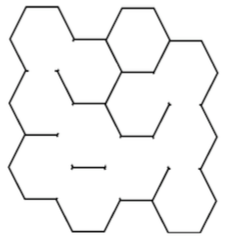Hamilton is a brave bee living in a general honeycomb. Now he wants to move from a starting point to a specified destination. The image below gives a feasible path in a $3 \times 4$ honeycomb from the $1$-st cell in the $2$-nd column to the $1$-st cell in the $4$-th column.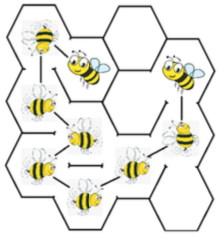Please help him find the minimum number of cells that a feasible path has to pass through (including the starting point and the destination) from the specified starting point to the destination.

## Input:

The input contains several test cases, and the first line contains a positive integer $T$ indicating the number of test cases which is up to $10^4$.
For each test case, the first line contains two integers $r$ and $c$ indicating the number of rows and the number of columns of the honeycomb, where $2 \leq r, c \leq 10^3$.
The following $(4 r + 3)$ lines describe the whole given honeycomb, where each line contains at most $(6 c + 3)$ characters. Odd lines contain grid vertices represented as plus signs ("+") and zero or more horizontal edges, while even lines contain two or more diagonal edges. Specifically, a cell is described as $6$ vertices and at most $6$ edges. Its upper boundary or lower boundary is represented as three consecutive minus signs ("-"). Each one of its diagonal edges, if exists, is a single forward slash ("/") or a single backslash ("") character. All edge characters will be placed exactly between the corresponding vertices. At the center of the starting cell (resp. the destination), a capital "S" (resp. a capital "T") as a special character is used to indicate the special cell. All other characters will be space characters. Note that if any input line could contain trailing whitespace, that whitespace will be omitted.
We guarantee that all outermost wall exist so that the given honeycomb is closed, and exactly one "S" and one "T" appear in the given honeycomb. Besides, the sum of $r \cdot c$ in all test cases is up to $2 \times 10^6$.

## Output:

For each test case, output a line containing the minimum number of cells that Hamilton has to visit moving from the starting cell ("S") to the destination ("T"), including the starting cell and the destination. If no feasible path exists, output -1 instead.

## Sample Input:

1
3 4
+---+       +---+
/          /     \
+       +---+       +---+
/     \
+   +   S   +---+   T   +
/          /           /
+       +---+       +   +
/     \
+---+       +---+       +
/                       /
+       +---+       +   +
/     \
+---+       +---+       +
/          /
+---+       +---+


## Sample Output:

7

### 题目链接

1G内存限制！！！数组随便开就好了。

memset会超时！

## AC代码:

#include <bits/stdc++.h>
using namespace std;

const int INF = 0x3f3f3f3f;
const int maxn = 1e4 + 5;

struct Status {
int X, Y, Step;
};

int T;
int R, C;
int N, M;
int Len;
int StartX, StartY;
int EndX, EndY;
char Plat[maxn][maxn];
int Vis[maxn][maxn];
int DX = {1, 1, -1, -1, 2, -2};
int DY = {3, -3, 3, -3, 0, 0};

int Bfs() {
queue<Status> Que;
Que.push(Status {StartX, StartY, 1});
Vis[StartX][StartY] = 0;
while (!Que.empty()) {
Status Cur = Que.front(); Que.pop();
if (Cur.X == EndX && Cur.Y == EndY) {
return Cur.Step;
}
for (int i = 0; i < 6; ++i) {
int NX = Cur.X + DX[i], NY = Cur.Y + DY[i];
if (NX >= 0 && NX < N && NY >= 0 && NY < M && Plat[NX][NY] == ' ') {
int NNX = NX + DX[i], NNY = NY + DY[i];
if (NNX >= 0 && NNX < N && NNY >= 0 && NNY < M && Cur.Step + 1 < Vis[NNX][NNY]) {
Que.push(Status {NNX, NNY, Cur.Step + 1});
Vis[NNX][NNY] = Cur.Step + 1;
}
}
}
}
return -1;
}

int main(int argc, char *argv[]) {
scanf("%d", &T);
for (int Case = 1; Case <= T; ++Case) {
scanf("%d%d", &R, &C);
N = 4 * R + 3, M = 6 * C + 3;
getchar();
for (int i = 0; i < N; ++i) {
fgets(Plat[i], maxn, stdin);
Len = strlen(Plat[i]);
if (Plat[i][Len - 1] == '\n') {
Plat[i][Len - 1] = '\0';
}
for (int j = 0; j < M; ++j) {
Vis[i][j] = INF;
if (Plat[i][j] == 'S') {
StartX = i; StartY = j;
}
if (Plat[i][j] == 'T') {
EndX = i; EndY = j;
}
}
}
printf("%d\n", Bfs());
}
return 0;
}

# I. Distance

## Description:

There are $n$ points on a horizontal line, labelled with $1$ through $n$ from left to right.
The distance between the $i$-th point and the $(i + 1)$-th point is $a_i$.
For each integer $k$ ranged from $1$ to $n$, you are asked to select exactly $k$ different given points on the line to maximize the sum of distances between all pairs of selected points.

## Input:

The input contains several test cases, and the first line contains a positive integer $T$ indicating the number of test cases which is up to $1000$.
For each test case, the first line contains an integer $n$ indicating the number of points, where $2 \leq n \leq 10^5$.
The second line contains $(n - 1)$ positive integers $a_1, a_2, \cdots, a_{n - 1}$, where $1 \leq a_i \leq 10^4$.
We guarantee that the sum of $n$ in all test cases is up to $10^6$.

## Output:

For each test case, output a line containing $n$ integers, the $i$-th of which is the maximum sum of distances in case $k = i$. You should output exactly one whitespace between every two adjacent numbers and avoid any trailing whitespace in this line.

1
5
2 3 1 4

0 10 20 34 48

## AC代码:

#include <bits/stdc++.h>
using namespace std;

const int maxn = 1e5 + 5;

long long T;
long long N;
long long Left, Right;
long long Cur;
long long Ans;
long long Pos[maxn];

int main(int argc, char *argv[]) {
scanf("%lld", &T);
for (long long Case = 1; Case <= T; ++Case) {
if (Case != 1) {
printf("\n");
}
scanf("%lld", &N);
Pos = 0;
for (long long i = 2, X; i <= N; ++i) {
scanf("%lld", &X);
Pos[i] = Pos[i - 1] + X;
}
Left = 0; Right = N + 1;
Ans = 0; Cur = 0;
for (int i = 1; i <= N; ++i) {
if (i & 1) {
Left++;
Ans += Cur;
}
else {
Right--;
Cur += Pos[Right] - Pos[Left];
Ans += Cur;
}
if (i != 1) {
printf(" ");
}
printf("%lld", Ans);
}
}
return 0;
}
Last modification：March 28th, 2019 at 12:46 am